# Concept: Time Series Data Types and Formats¶

This lesson covers the different types of time series datasets and the formats in which they can be stored.## Types of Time Series Data¶

Recall that a time series dataset can contain one or more variables of an entity repeatedly measured over time. Depending on the number of variables in a time series, and the relationships between the variables, time series data can be categorized as:

• Univariate

• Multivariate

• Multiple

### Univariate¶

A univariate time series consists of sequential measurements of a single variable over time. Consider a time series dataset that contains measurements of a person named Mike, who has certain features (or variables), such as gender, height, weight, and pulse. If we collect measurements of one of these variables, say Mike’s weight, over time, we have a univariate time series.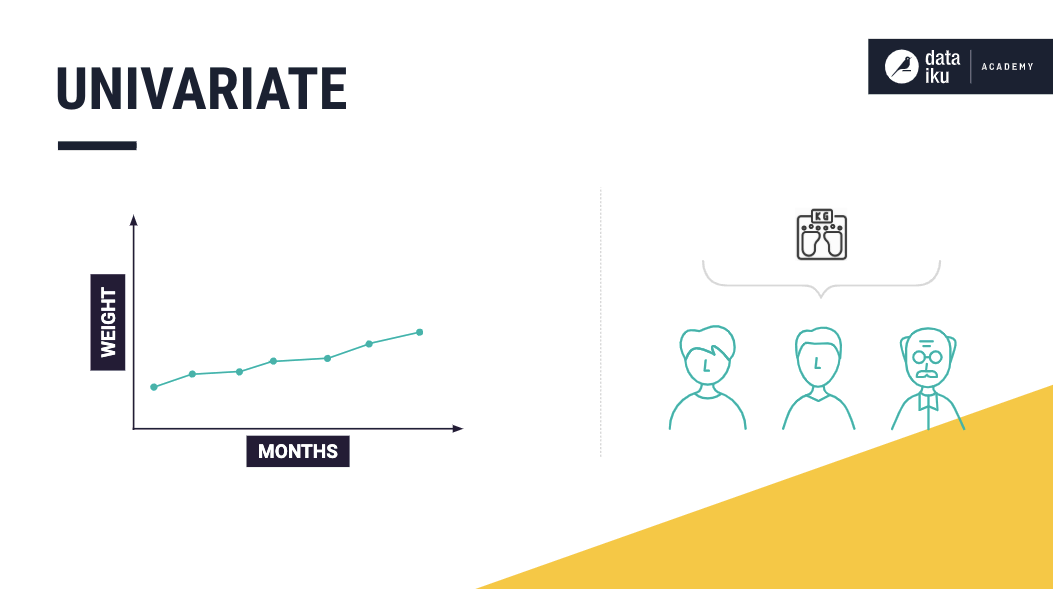Using these values of Mike’s weight, we can build a model to predict his future weight.

### Multivariate¶

A multivariate time series consists of sequential measurements of multiple related variables over time. For example, suppose our data set consists of the measurements of Mike’s height and weight, and we know that there is a relationship between the two variables (weight and height). In that case, we have a multivariate time series. Or, more specifically, a bivariate time series. This is because our dataset consists of exactly two variables that are interrelated.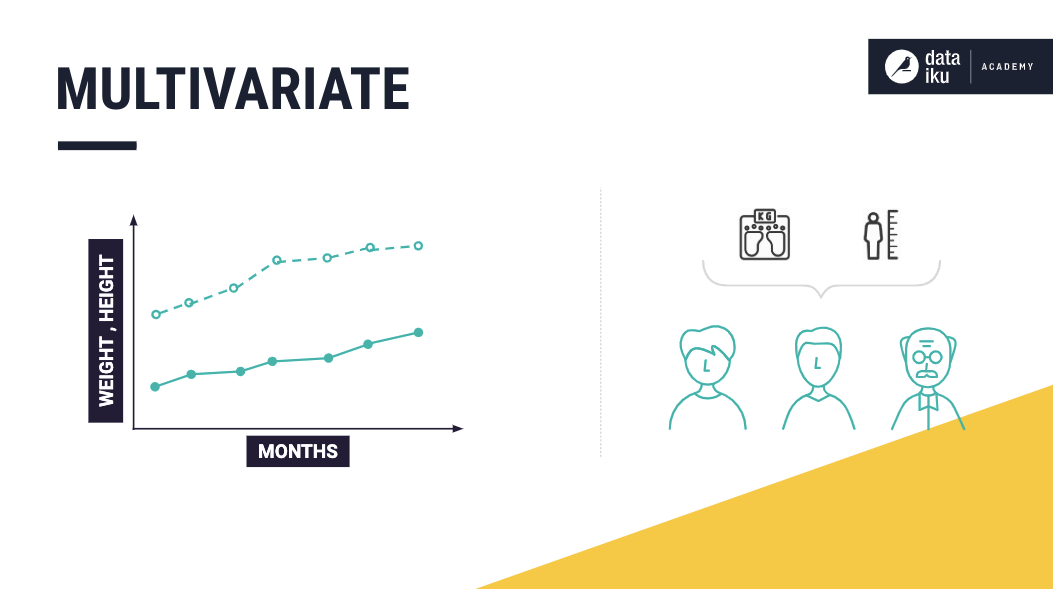Using these values of Mike’s weight and height, we can build a prediction model to determine his future weight or height.

### Multiple¶

A time series data set is said to contain multiple time series if it contains measurements of multiple entities that are independent. Now, let’s build upon the univariate example, by including measurements about Mike’s neighbor’s, Kate’s, weight. Suppose we know that the measurements of these individuals are independent of each other. In that case, we can say that the dataset contains multiple univariate time series, and predicting the weight of an individual would depend on his or her previous weights alone.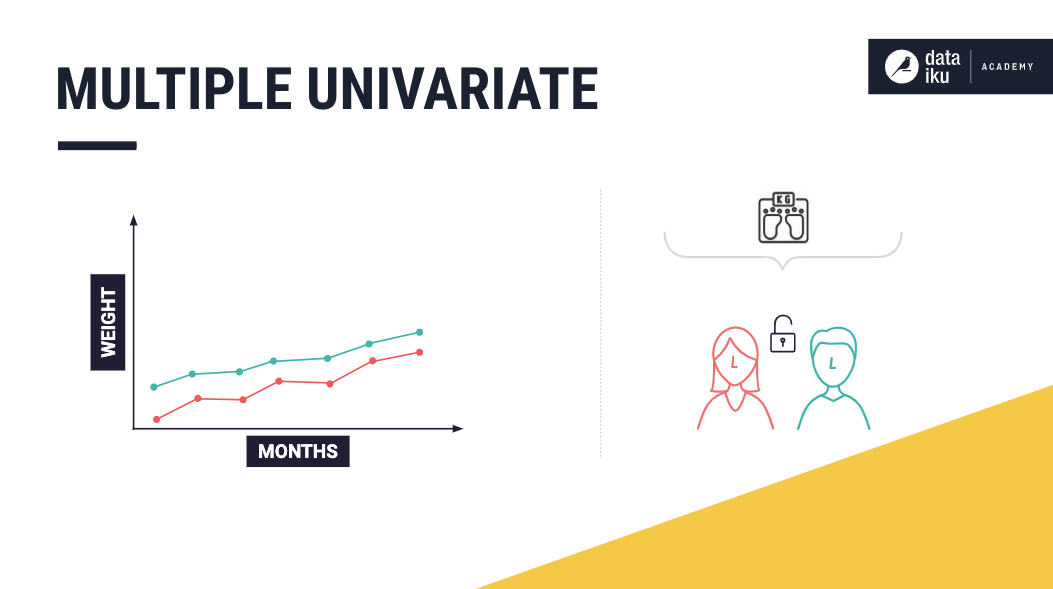Furthermore, if the dataset also contains the heights of these two individuals, then we have multiple multivariate time series in our dataset.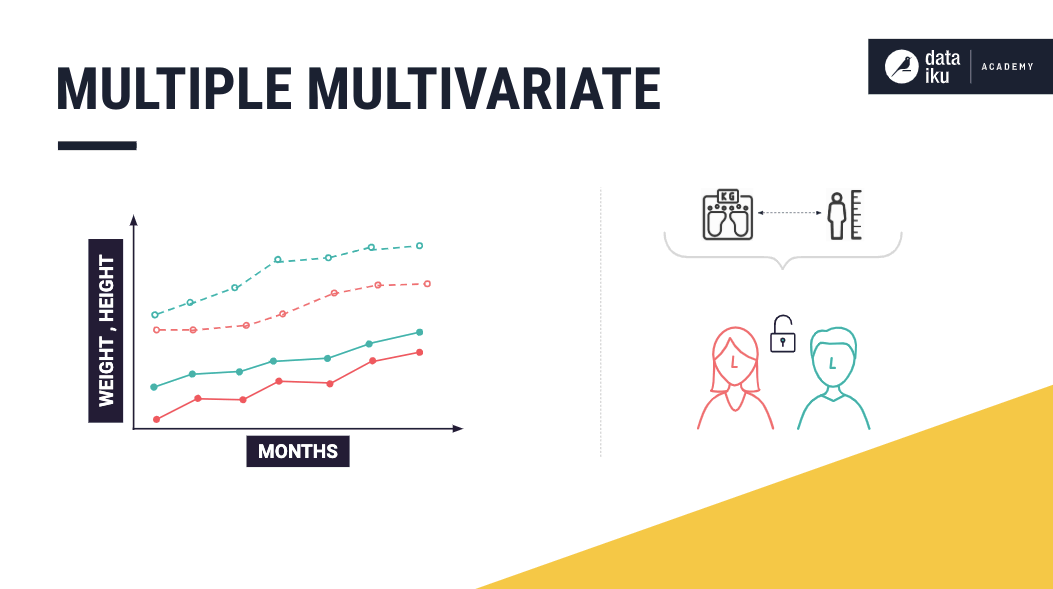## Time Series Data Formats¶

Dataiku DSS works with time series datasets that come in wide format or long format.

### Wide Format¶

To explain the wide format, consider the case where the time series dataset consists of multiple univariate time series — the weights for Mike and Kate. This dataset is in wide format if each univariate time series is stored in a separate column.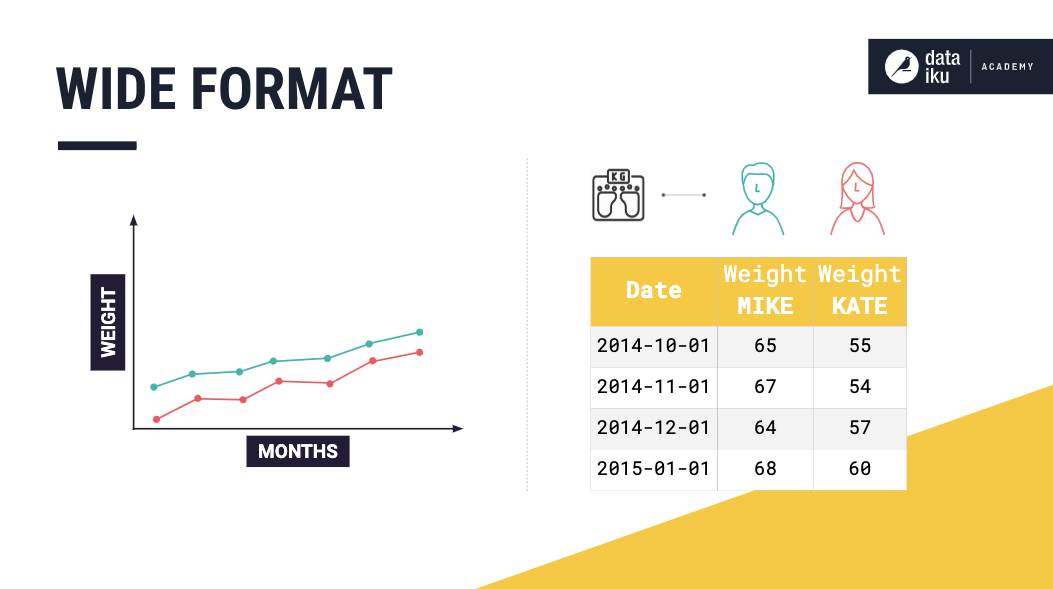Furthermore, the dataset could contain multiple multivariate time series. Such as the measurements of Mike’s height and weight — a multivariate time series and the measurements of Kate’s height and weight — another multivariate time series.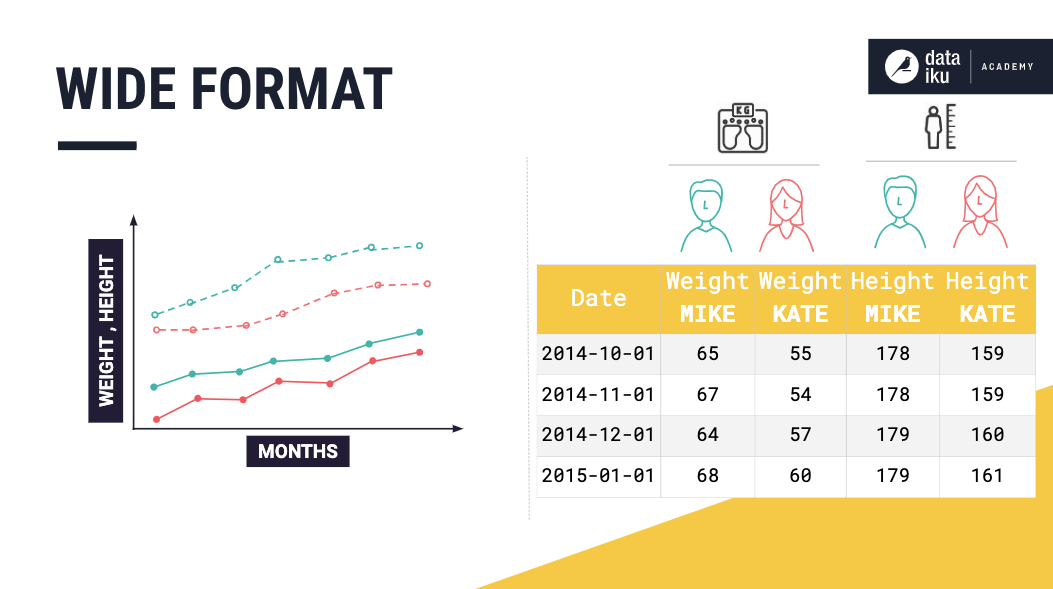Wide format representation is easy to understand and more natural to use when plotting. However, using this format can present issues when there are missing values in the data. For example, if Mike decides to drop out of this experiment, we must decide whether to keep adding empty rows for Mike’s measurements.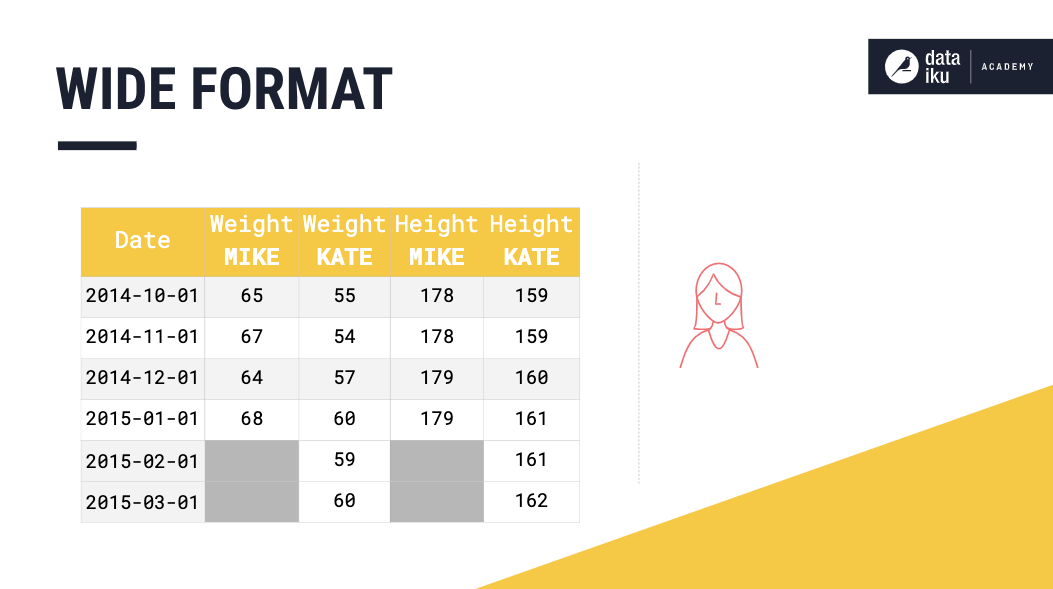Or whether to drop Mike’s columns entirely.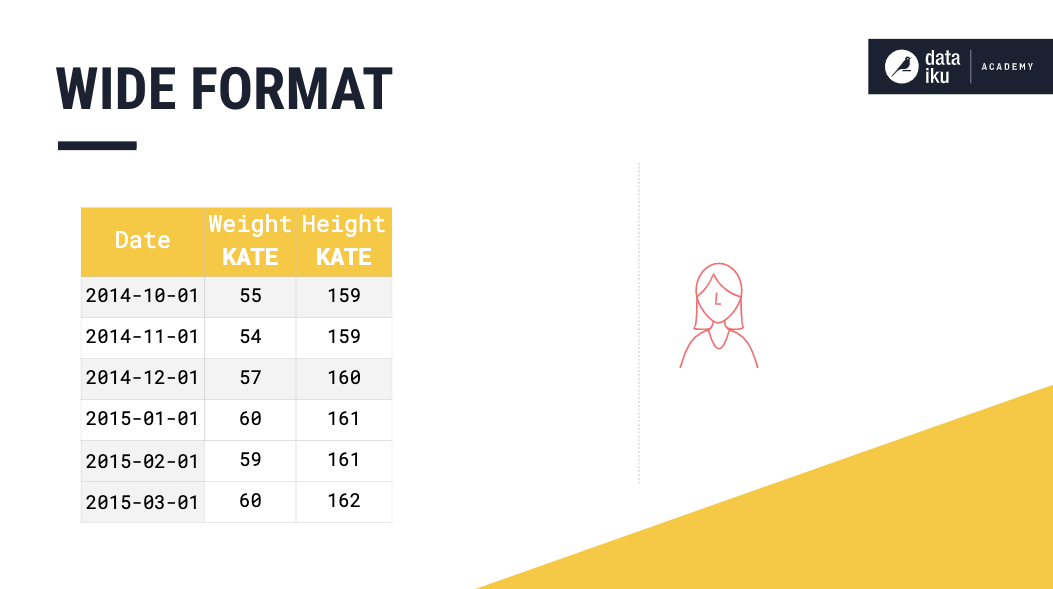### Long Format¶

This format provides a compact way of representing multiple time series. Consider a time series dataset that consists of multiple univariate time series. In long format, values from the univariate time series are all stored in the same column.

Storing the data this way makes it necessary to have an identifier column that tells us which time series each row belongs to.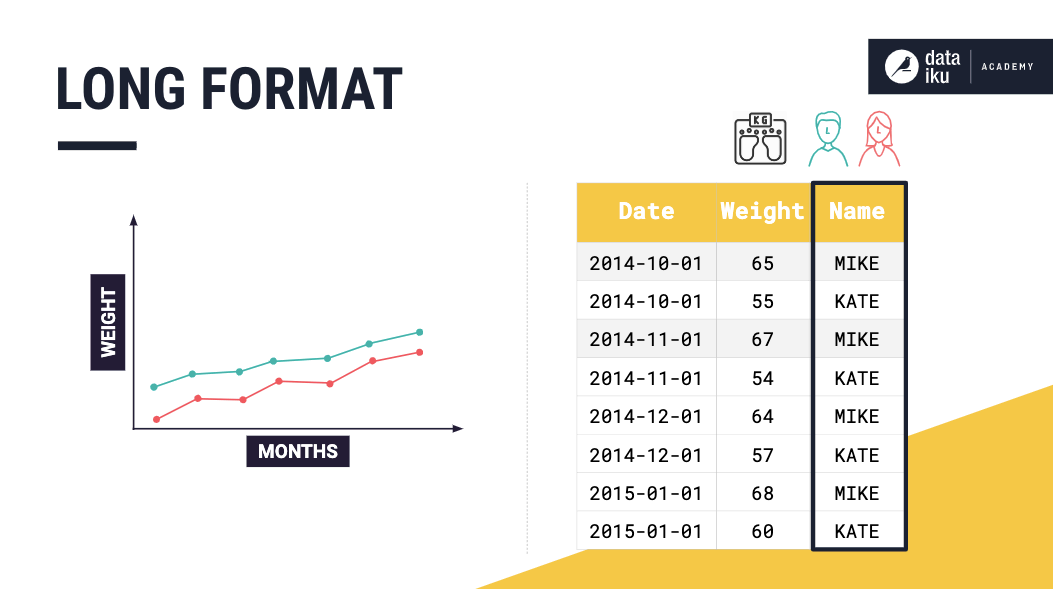A multivariate time series dataset can also be stored in long format, and in this case, the identifier column will list the variables of a given entity.

Using the long format can provide a more compact way to represent time series datasets when compared to the wide format. For illustration, consider that we have the weights for Mike and Kate, and we decide to start measuring Jon’s weight as well. Using long format, we would simply add a new row for Jon. Whereas, if our dataset is in wide format, we would have to add a new column for Jon, and fill in missing values for the dates before we made Jon’s first measurement.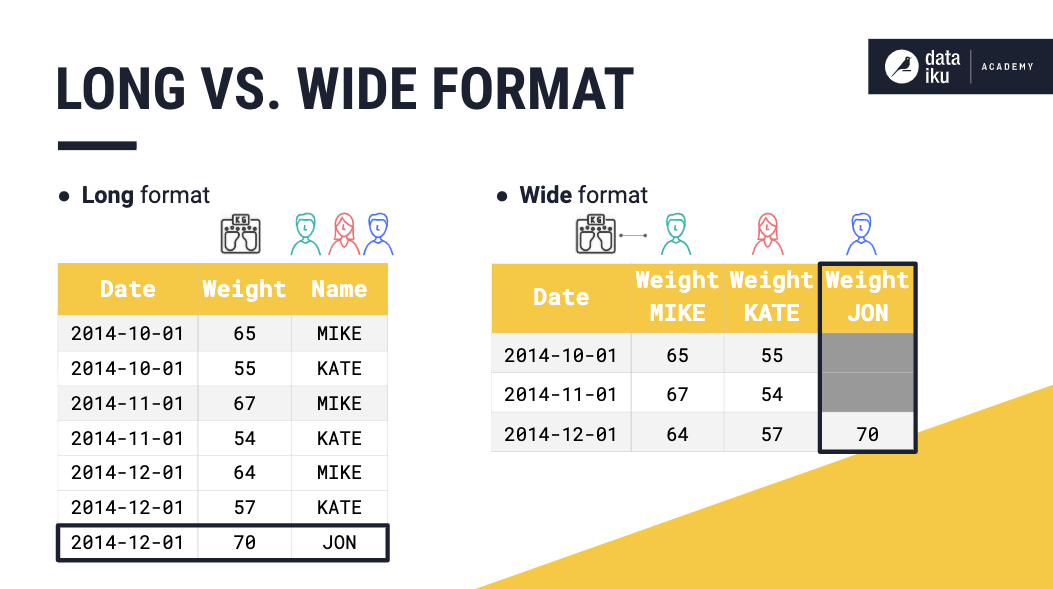### Long Vs. Wide Format¶

Often, the choice of which format to use in storing time series data sets depends on the kinds of models that will be used on them. For example, the wide format may be more suitable for analyses like MANOVA and repeated measures ANOVA. On the other hand, if we are interested in mixed models or survival analysis, using long format may be more appropriate.